# 共振补偿¶

Klipper支持输入整形 -一种可以用来减少打印件上振纹（也被称为echo、ghosting或ripping）的技术。振纹是一种表面打印缺陷，通常在边角的位置表面重复出现，成为一种微妙的水波状纹路：

|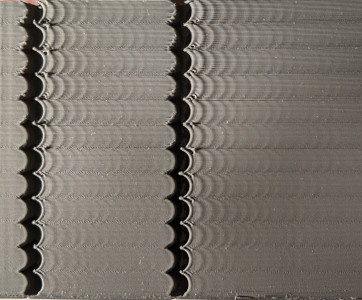|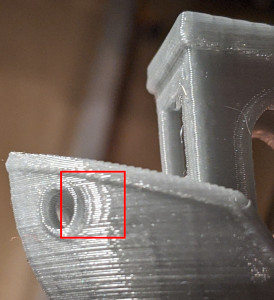|

## 调整¶

Basic tuning requires measuring the ringing frequencies of the printer by printing a test model.

• 建议将层高为 0.2 或 0.25 毫米。
• 填充和顶层层数可以被设置为0。
• 使用1-2圈壁，使用1-2毫米厚底坐和花瓶模式(vasemode)的效果更好。
• 使用足够高的速度，大约80-100毫米/秒，用于外部周长（壁）。
• 确保最短的层耗时最多是3秒。
• 确保切片软件中禁用任何"动态加速度控制"功能。
• 不要转动模型。模型的背面标记了X和Y。注意这些标记与打印机轴线方向不相同--这不是一个错误。这些标记可以在以后的调整过程中作为参考，因为它们显示了测量结果对应的轴。

### 振纹频率¶

1. If `square_corner_velocity` parameter was changed, revert it back to 5.0. It is not advised to increase it when using input shaper because it can cause more smoothing in parts - it is better to use higher acceleration value instead.
2. Increase `max_accel_to_decel` by issuing the following command: `SET_VELOCITY_LIMIT ACCEL_TO_DECEL=7000`
3. Disable Pressure Advance: `SET_PRESSURE_ADVANCE ADVANCE=0`
4. 如果你已经将`[input_shaper]`分段添加到print.cfg中，执行`SET_INPUT_SHAPER SHAPER_FREQ_X=0 SHAPER_FREQ_Y=0`命令。如果你得到"未知命令"错误，此时你可以安全地忽略它，继续进行测量。
5. Execute the command: `TUNING_TOWER COMMAND=SET_VELOCITY_LIMIT PARAMETER=ACCEL START=1500 STEP_DELTA=500 STEP_HEIGHT=5` Basically, we try to make ringing more pronounced by setting different large values for acceleration. This command will increase the acceleration every 5 mm starting from 1500 mm/sec^2: 1500 mm/sec^2, 2000 mm/sec^2, 2500 mm/sec^2 and so forth up until 7000 mm/sec^2 at the last band.
6. 打印用建议的参数切片的测试模型。
7. 如果振纹清晰可见，并且发现加速度对你的打印机来说太高了（如打印机抖动太厉害或开始丢步），你可以提前停止打印。

1. 使用模型后侧的XY标志作为校准的参考。X标志所在一侧的测量结果用于X轴的配置，而Y标志所在一侧则作为Y轴的配置。以X轴为例，测量X标志所在一侧的数个振纹周期的长度D（单位mm）：首先确定凹槽的位置，为了测量准确可以忽略最靠近凹槽的数个纹路，用记号笔标记起始和结束的数个振纹，然后用尺子或卡尺进行测量：|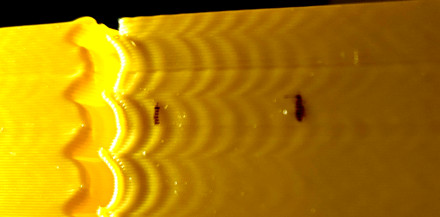|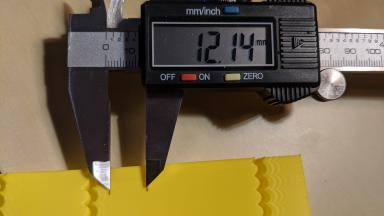|
2. 数一数测量的距离D中有多少个振荡D。如果你不确定如何计算振荡，请参考上图，其中显示N=6次振荡。
3. 通过 V · N / D (Hz) 计算振铃的频率，其中V 是外壁的加速度（mm/秒）。在上述例子中，我们标记了6个振纹，同时测试件是以100 mm/秒的速度进行印制，因此振动频率为100 * 6 / 12.14 ≈ 49.4 Hz。
4. Do (8) - (10) for Y mark as well.

• 安装、移除、更换了一些在打印头上的工具，从而改变了其质量，例如，为近程挤出机更换一个新的（更重或更轻的）步进电机，或安装一个新的热端，增加一个带风道的重型风扇，等等。
• 同步带被拉紧。
• 安装了一些增加框架刚性的配件。
• 对平移热床式打印机而言，使用了不同的热床面板，或者加装了玻璃面板等操作。

### 输入整形器配置¶

``````[input_shaper]
shaper_freq_x: ...  # frequency for the X mark of the test model
shaper_freq_y: ...  # frequency for the Y mark of the test model
``````

### 选择输入整形器¶

Klipper支持数种输入整形器。这些整形器之间的差异在于它们容许的共振频率测量误差和它们在打印件中产生的平滑度。请注意，一些整形器，例如2HUMP_EI和3HUMP_EI，不应与shaper_freq = 共振频率一起使用。它们应该被配置为同时减少多个不同的频率。

Print the ringing test model as follows:

1. Restart the firmware: `RESTART`
2. Prepare for test: `SET_VELOCITY_LIMIT ACCEL_TO_DECEL=7000`
3. Disable Pressure Advance: `SET_PRESSURE_ADVANCE ADVANCE=0`
4. Execute: `SET_INPUT_SHAPER SHAPER_TYPE=MZV`
5. Execute the command: `TUNING_TOWER COMMAND=SET_VELOCITY_LIMIT PARAMETER=ACCEL START=1500 STEP_DELTA=500 STEP_HEIGHT=5`
6. 打印用建议的参数切片的测试模型。

Now try EI input shaper. To try it, repeat steps (1)-(6) from above, but executing at step 4 the following command instead: `SET_INPUT_SHAPER SHAPER_TYPE=EI`.

``````[input_shaper]
shaper_freq_x: ...
shaper_freq_y: ...
shaper_type: mzv
``````

• EI 整形器可能更适用于平移热床的打印机（如果共振频率和由此产生的平滑度允许）：随着更多的耗材被打印到在移动的打印床上，床的质量增加，造成共振频率降低。由于 EI 整形器对共振频率的变化更为稳健，在打印大型部件时可能效果更好。
• 由于三角洲运动学的性质，共振频率在可打印范围内不同位置会有很大的不同。因此，EI整形器可以更好地适用于三角洲打印机，而不是MZV或ZV，应该考虑使用。如果共振频率足够大（超过50-60赫兹），那么甚至可以尝试测试2HUMP_EI整形器（通过运行上面建议的测试`SET_INPUT_SHAPER SHAPER_TYPE=2HUMP_EI`），但在启用之前要检查下面的章节的注意事项。

### 选择 max_accel¶

You should have a printed test for the shaper you chose from the previous step (if you don't, print the test model sliced with the suggested parameters with the pressure advance disabled `SET_PRESSURE_ADVANCE ADVANCE=0` and with the tuning tower enabled as `TUNING_TOWER COMMAND=SET_VELOCITY_LIMIT PARAMETER=ACCEL START=1500 STEP_DELTA=500 STEP_HEIGHT=5`). Note that at very high accelerations, depending on the resonance frequency and the input shaper you chose (e.g. EI shaper creates more smoothing than MZV), input shaping may cause too much smoothing and rounding of the parts. So, max_accel should be chosen such as to prevent that. Another parameter that can impact smoothing is `square_corner_velocity`, so it is not advisable to increase it above the default 5 mm/sec to prevent increased smoothing.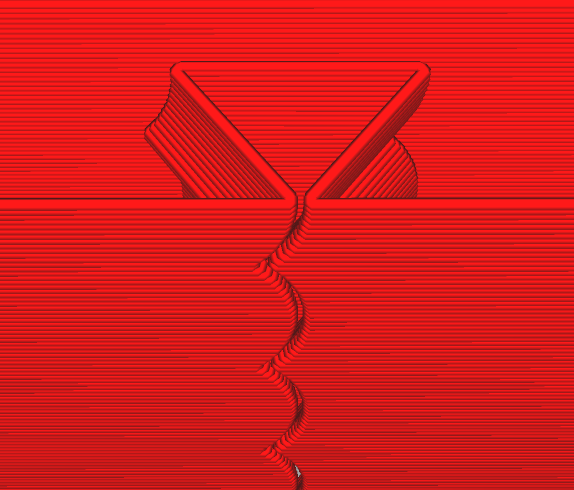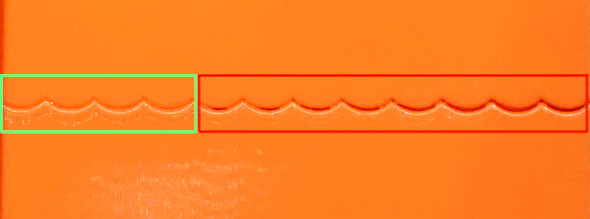Choose the minimum out of the two acceleration values (from ringing and smoothing), and put it as `max_accel` into printer.cfg.

Another consideration is that if a resonance frequency is too low (below 20-25 Hz), it might be a good idea to increase the printer stiffness or reduce the moving mass. Otherwise, acceleration and printing speed may be limited due too much smoothing now instead of ringing.

### Fine-tuning resonance frequencies¶

Note that the precision of the resonance frequencies measurements using the ringing test model is sufficient for most purposes, so further tuning is not advised. If you still want to try to double-check your results (e.g. if you still see some ringing after printing a test model with an input shaper of your choice with the same frequencies as you have measured earlier), you can follow the steps in this section. Note that if you see ringing at different frequencies after enabling [input_shaper], this section will not help with that.

Assuming that you have sliced the ringing model with suggested parameters, complete the following steps for each of the axes X and Y:

1. Prepare for test: `SET_VELOCITY_LIMIT ACCEL_TO_DECEL=7000`
2. Make sure Pressure Advance is disabled: `SET_PRESSURE_ADVANCE ADVANCE=0`
3. Execute: `SET_INPUT_SHAPER SHAPER_TYPE=ZV`
4. From the existing ringing test model with your chosen input shaper select the acceleration that shows ringing sufficiently well, and set it with: `SET_VELOCITY_LIMIT ACCEL=...`
5. Calculate the necessary parameters for the `TUNING_TOWER` command to tune `shaper_freq_x` parameter as follows: start = shaper_freq_x * 83 / 132 and factor = shaper_freq_x / 66, where `shaper_freq_x` here is the current value in `printer.cfg`.
6. Execute the command: `TUNING_TOWER COMMAND=SET_INPUT_SHAPER PARAMETER=SHAPER_FREQ_X START=start FACTOR=factor BAND=5` using `start` and `factor` values calculated at step (5).
7. Print the test model.
8. Reset the original frequency value: `SET_INPUT_SHAPER SHAPER_FREQ_X=...`.
9. Find the band which shows ringing the least and count its number from the bottom starting at 1.
10. Calculate the new shaper_freq_x value via old shaper_freq_x * (39 + 5 * #band-number) / 66.

Repeat these steps for the Y axis in the same manner, replacing references to X axis with the axis Y (e.g. replace `shaper_freq_x` with `shaper_freq_y` in the formulae and in the `TUNING_TOWER` command).

As an example, let's assume you have had measured the ringing frequency for one of the axis equal to 45 Hz. This gives start = 45 * 83 / 132 = 28.30 and factor = 45 / 66 = 0.6818 values for `TUNING_TOWER` command. Now let's assume that after printing the test model, the fourth band from the bottom gives the least ringing. This gives the updated shaper_freq_? value equal to 45 * (39 + 5 * 4) / 66 ≈ 40.23.

After both new `shaper_freq_x` and `shaper_freq_y` parameters have been calculated, you can update `[input_shaper]` section in `printer.cfg` with the new `shaper_freq_x` and `shaper_freq_y` values.

If you use Pressure Advance, it may need to be re-tuned. Follow the instructions to find the new value, if it differs from the previous one. Make sure to restart Klipper before tuning Pressure Advance.

### Unreliable measurements of ringing frequencies¶

If you are unable to measure the ringing frequencies, e.g. if the distance between the oscillations is not stable, you may still be able to take advantage of input shaping techniques, but the results may not be as good as with proper measurements of the frequencies, and will require a bit more tuning and printing the test model. Note that another possibility is to purchase and install an accelerometer and measure the resonances with it (refer to the docs describing the required hardware and the setup process) - but this option requires some crimping and soldering.

For tuning, add empty `[input_shaper]` section to your `printer.cfg`. Then, assuming that you have sliced the ringing model with suggested parameters, print the test model 3 times as follows. First time, prior to printing, run

1. `RESTART`
2. `SET_VELOCITY_LIMIT ACCEL_TO_DECEL=7000`
3. `SET_PRESSURE_ADVANCE ADVANCE=0`
4. `SET_INPUT_SHAPER SHAPER_TYPE=2HUMP_EI SHAPER_FREQ_X=60 SHAPER_FREQ_Y=60`
5. `TUNING_TOWER COMMAND=SET_VELOCITY_LIMIT PARAMETER=ACCEL START=1500 STEP_DELTA=500 STEP_HEIGHT=5`

and print the model. Then print the model again, but before printing run instead

1. `SET_INPUT_SHAPER SHAPER_TYPE=2HUMP_EI SHAPER_FREQ_X=50 SHAPER_FREQ_Y=50`
2. `TUNING_TOWER COMMAND=SET_VELOCITY_LIMIT PARAMETER=ACCEL START=1500 STEP_DELTA=500 STEP_HEIGHT=5`

Then print the model for the 3rd time, but now run

1. `SET_INPUT_SHAPER SHAPER_TYPE=2HUMP_EI SHAPER_FREQ_X=40 SHAPER_FREQ_Y=40`
2. `TUNING_TOWER COMMAND=SET_VELOCITY_LIMIT PARAMETER=ACCEL START=1500 STEP_DELTA=500 STEP_HEIGHT=5`

Essentially, we are printing the ringing test model with TUNING_TOWER using 2HUMP_EI shaper with shaper_freq = 60 Hz, 50 Hz, and 40 Hz.

If none of the models demonstrate improvements in ringing, then, unfortunately, it does not look like the input shaping techniques can help with your case.

Otherwise, it may be that all models show no ringing, or some show the ringing and some - not so much. Choose the test model with the highest frequency that still shows good improvements in ringing. For example, if 40 Hz and 50 Hz models show almost no ringing, and 60 Hz model already shows some more ringing, stick with 50 Hz.

Now check if EI shaper would be good enough in your case. Choose EI shaper frequency based on the frequency of 2HUMP_EI shaper you chose:

• For 2HUMP_EI 60 Hz shaper, use EI shaper with shaper_freq = 50 Hz.
• For 2HUMP_EI 50 Hz shaper, use EI shaper with shaper_freq = 40 Hz.
• For 2HUMP_EI 40 Hz shaper, use EI shaper with shaper_freq = 33 Hz.

Now print the test model one more time, running

1. `SET_INPUT_SHAPER SHAPER_TYPE=EI SHAPER_FREQ_X=... SHAPER_FREQ_Y=...`
2. `TUNING_TOWER COMMAND=SET_VELOCITY_LIMIT PARAMETER=ACCEL START=1500 STEP_DELTA=500 STEP_HEIGHT=5`

providing the shaper_freq_x=... and shaper_freq_y=... as determined previously.

If EI shaper shows very comparable good results as 2HUMP_EI shaper, stick with EI shaper and the frequency determined earlier, otherwise use 2HUMP_EI shaper with the corresponding frequency. Add the results to `printer.cfg` as, e.g.

``````[input_shaper]
shaper_freq_x: 50
shaper_freq_y: 50
shaper_type: 2hump_ei
``````

Continue the tuning with Selecting max_accel section.

## Troubleshooting and FAQ¶

### I cannot get reliable measurements of resonance frequencies¶

First, make sure it is not some other problem with the printer instead of ringing. If the measurements are not reliable because, say, the distance between the oscillations is not stable, it might mean that the printer has several resonance frequencies on the same axis. One may try to follow the tuning process described in Unreliable measurements of ringing frequencies section and still get something out of the input shaping technique. Another possibility is to install an accelerometer, measure the resonances with it, and auto-tune the input shaper using the results of those measurements.

### After enabling [input_shaper], I get too smoothed printed parts and fine details are lost¶

Check the considerations in Selecting max_accel section. If the resonance frequency is low, one should not set too high max_accel or increase square_corner_velocity parameters. It might also be better to choose MZV or even ZV input shapers over EI (or 2HUMP_EI and 3HUMP_EI shapers).

### After successfully printing for some time without ringing, it appears to come back¶

It is possible that after some time the resonance frequencies have changed. E.g. maybe the belts tension has changed (belts got more loose), etc. It is a good idea to check and re-measure the ringing frequencies as described in Ringing frequency section and update your config file if necessary.

### Is dual carriage setup supported with input shapers?¶

There is no dedicated support for dual carriages with input shapers, but it does not mean this setup will not work. One should run the tuning twice for each of the carriages, and calculate the ringing frequencies for X and Y axes for each of the carriages independently. Then put the values for carriage 0 into [input_shaper] section, and change the values on the fly when changing carriages, e.g. as a part of some macro:

``````SET_DUAL_CARRIAGE CARRIAGE=1
SET_INPUT_SHAPER SHAPER_FREQ_X=... SHAPER_FREQ_Y=...
``````

And similarly when switching back to carriage 0.

### Does input_shaper affect print time?¶

No, `input_shaper` feature has pretty much no impact on the print times by itself. However, the value of `max_accel` certainly does (tuning of this parameter described in this section).

## Technical details¶

### Input shapers¶

Input shapers used in Klipper are rather standard, and one can find more in-depth overview in the articles describing the corresponding shapers. This section contains a brief overview of some technical aspects of the supported input shapers. The table below shows some (usually approximate) parameters of each shaper.

Input
shaper
Shaper
duration
Vibration reduction 20x
(5% vibration tolerance)
Vibration reduction 10x
(10% vibration tolerance)
ZV 0.5 / shaper_freq N/A ± 5% shaper_freq
MZV 0.75 / shaper_freq ± 4% shaper_freq -10%...+15% shaper_freq
ZVD 1 / shaper_freq ± 15% shaper_freq ± 22% shaper_freq
EI 1 / shaper_freq ± 20% shaper_freq ± 25% shaper_freq
2HUMP_EI 1.5 / shaper_freq ± 35% shaper_freq ± 40 shaper_freq
3HUMP_EI 2 / shaper_freq -45...+50% shaper_freq -50%...+55% shaper_freq

A note on vibration reduction: the values in the table above are approximate. If the damping ratio of the printer is known for each axis, the shaper can be configured more precisely and it will then reduce the resonances in a bit wider range of frequencies. However, the damping ratio is usually unknown and is hard to estimate without a special equipment, so Klipper uses 0.1 value by default, which is a good all-round value. The frequency ranges in the table cover a number of different possible damping ratios around that value (approx. from 0.05 to 0.2).

Also note that EI, 2HUMP_EI, and 3HUMP_EI are tuned to reduce vibrations to 5%, so the values for 10% vibration tolerance are provided only for the reference.

How to use this table:

• Shaper duration affects the smoothing in parts - the larger it is, the more smooth the parts are. This dependency is not linear, but can give a sense of which shapers 'smooth' more for the same frequency. The ordering by smoothing is like this: ZV < MZV < ZVD ≈ EI < 2HUMP_EI < 3HUMP_EI. Also, it is rarely practical to set shaper_freq = resonance freq for shapers 2HUMP_EI and 3HUMP_EI (they should be used to reduce vibrations for several frequencies).
• One can estimate a range of frequencies in which the shaper reduces vibrations. For example, MZV with shaper_freq = 35 Hz reduces vibrations to 5% for frequencies [33.6, 36.4] Hz. 3HUMP_EI with shaper_freq = 50 Hz reduces vibrations to 5% in range [27.5, 75] Hz.
• One can use this table to check which shaper they should be using if they need to reduce vibrations at several frequencies. For example, if one has resonances at 35 Hz and 60 Hz on the same axis: a) EI shaper needs to have shaper_freq = 35 / (1 - 0.2) = 43.75 Hz, and it will reduce resonances until 43.75 * (1 + 0.2) = 52.5 Hz, so it is not sufficient; b) 2HUMP_EI shaper needs to have shaper_freq = 35 / (1 - 0.35) = 53.85 Hz and will reduce vibrations until 53.85 * (1 + 0.35) = 72.7 Hz - so this is an acceptable configuration. Always try to use as high shaper_freq as possible for a given shaper (perhaps with some safety margin, so in this example shaper_freq ≈ 50-52 Hz would work best), and try to use a shaper with as small shaper duration as possible.
• If one needs to reduce vibrations at several very different frequencies (say, 30 Hz and 100 Hz), they may see that the table above does not provide enough information. In this case one may have more luck with scripts/graph_shaper.py script, which is more flexible.